# Background

Let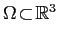be a bounded, simply or multiply connected domain in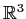with a Lipschitz boundary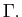The Laplace equation for a scalar function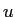in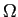is given by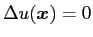or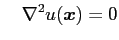or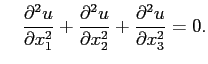A solutionof the Laplace equation admits an integral representation, known as the Green's representation formula, expressed as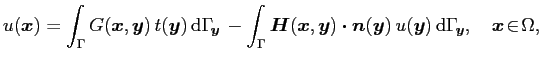where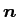is the unit normal to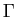directed towards the exterior of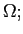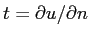is the flux associated with the potential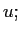and the kernels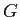and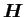are given respectively by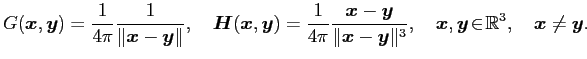Here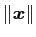is the usual Euclidean norm indefined as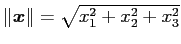.

# Solution via a boundary element method

To approximately solve the Laplace equation via a Boundary Element Method (BEM), the surfaceis usually discretized into flat triangles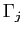using a mesh generation software (e.g. CUBIT).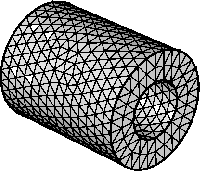With reference to , the potentialand flux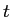are assumed to have a polynomial variation over each triangle (boundary element)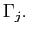# References

 S. Nintcheu Fata.
Explicit expressions for 3D boundary integrals in potential theory.
Int. J. Num. Meth. Eng., 78(1):32-47, 2009.